NEW
Materials and photos
are now available.

# Talks

## Alain Albouy On generation of independent quadratic conserved quantities

In a Hamiltonian system one can produce a conserved quantity from two conserved quantities by using the Poisson bracket. Jacobi considered this remark as the ``deepest discovery by Poisson", while other authors, as Bertrand, remarked that nobody ever discovered a new conserved quantity by using this process.
Hans Lundmark observed a more spectacular way of producing new conserved quantities from two given ones. With his advisor Stefan Rauch-Wojciechowski, they considered another class of equations, that they call the Newton systems, where, in a vector space of dimension \$n\$, a force depends on the position and defines the second derivative of the position with respect to time. Then two conserved quantities which are quadratic in the velocities produce n-2 other ones. The theorem also works on a spherical space. In the Neumann problem on an n-dimensional sphere, starting with the energy and another quadratic conserved quantity, one produces in this way a (known) system of n quadratic independent conserved quantities in involution.
Recently, we found with Lundmark a simple criterion for the functional independence of conserved quantities produced in such a way. We present the result quite simply, using the ``projective dynamics" point of view, i.e. the properties of central projection in dynamics discovered by Appell in 1890.
(joint work with Hans Lundmark)
See the slides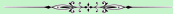## Elie Assémat Singularities of Hamiltonian integrable systems in physics

The phase space of integrable systems is foliated by tori, and some of them can be singular. The existence of these Hamiltonian singularities has been known for a few decades in mathematics, but their application in physics is very recent. This talk will first present one of the consequences of these singular tori: the Hamiltonian monodromy. This phenomenom can be surprisingly observed in physical PDEs. In nonlinear optics, the dynamics of some nonlinear waves systems relaxes towards a stationary state that possesses an integrable Hamiltonian structure. Finally, we will show that the singular tori of the Hamiltonian system coming from the Pontryagin's Maximum Principle is related to the STIRAP scheme, which is a control process well known for its robustness in quantum physics. This control process is commonly used to achieve a robust population transfer in low dimensional quantum systems.
See the slides## Boris Bardin Transcedental cases of the problem of stability in integrable and non-integrable hamiltonian systems

The talk will be about the use of an additional first integral for analysis of the stability of a periodic motion of a hamiltonian system in a particular case when the standard method of studying the stability, based on the analysis of a hamiltonian, normalized to the finite power terms, is not applicable. As an example I will consider the problem of stability of periodic motions of a rigid body in the Goryachev-Tchaplygin case. I will also comment on the general approach of stability analysis in the transcendental cases in the absence of an additional first integral.
See the slides## Bernard Bonnard Riemannian metrics on 2D-manifolds related to the Euler-Poinsot rigid body motion

The Euler-Poinsot rigid body motion is the model for the left-invariant Riemannian metrics on SO(3). In this talk we study two related metrics on 2D manifold: the metric of the Serret-Andoyer reduction and a metric associated to the dynamics of spin -1/2 particles. The conjugate and cut loci are discussed using both explicit and numerical computations as testbed of the Hampath code.
See the slides## Yuri Fedorov A puzzle of 3 Jacobians and separation of variables for some classical integrable systems.

It is known that complex invariant tori of several classical integrable systems, like the Clebsch case of the Kirchhoff equations, the Euler--Frahm top on SO(4) and the Kovalevskaya top are 2-dimensional Abelian (Prym) varieties with polarization (1,2), and they arise as subvarieties of Jacobians of a genus 3 curve (the spectral curve of the corresponding Lax representation).
On the other hand, more than a century ago, these systems were linearized by F. Koetter, H. Schottky, S. Kovalevskaya on Jacobians of genus 2 curves after intricate computations, whose algebraic geometric background is still discussed in the literature.
We contribute to this discussion by showing that in each case there are, in fact, 3 birationally non-equivalent hyperelliptic curves whose Jacobians are different 2-fold covering of the Prym variety. Thus, the above integrable systems can be linearized on any of the above Jacobians. Moreover, given the genus 3 spectral curve, all the three genus 2 curves can be written explicitly, which also allows to make explicitly 3 different separations of variables for each of the above systems.
In the talk all the notions will be explained and an explicit example will be considered.
The talk is based on results of a joint work with Viktor Enolski (Kiev).
See the slides## Jean-Pierre Francoise Analytic prolongation of normal forms for integrable Hamiltonian Systems

Perturbation theory of integrable systems displays locally convergent series. Different tools of complex analysis (relative cohomology, residue) are developed to yield analytic prolongation for these normal forms.## Božidar Jovanović Isoenergetic integrability and contact systems with constraints

We consider integrable Hamiltonian systems restricted to the hypersurfaces of the contact type and prove that the appropriate Reeb flows are examples of contact integrable systems. As a bi-product we obtain a partial version of the Arnold-Liouville theorem, where the system not need to be integrable on the whole phase space, while the invariant hypersurface is foliated on invariant Lagrangian tori. In the second part of the talk we consider contact systems with constraints. As an example, it is shown that the well known Reeb flows on Brieskorn manifolds are contact integrable in a non-commutative sense.
(joint work with Vladimir Jovanović, University of Banja Luka)
See the slides## Clémence Labrousse Complexity of integrable geodesic flows on tori

We look for the metrics on tori that minimize the entropy of the associated geodesic flow. Since the topological entropy may vanish, we study the polynomial entropy. We first see that flat metrics on Tn are minimizers for the polynomial entropy. Then we see that among a particular class of integrable geodesic flow on the torus T2, the flat metrics are strict local minimizers for the polynomial entropy.## Jean-Pierre Marco Some measurements of the complexity of integrable systems

We will introduce various measurements of the complexity of general dynamical systems (essentially based on an asymptotic expansion of the growth rate of characteristic quantities when the time tends to infinity) and we will examine their relevance when applied to specific classes of integrable hamiltonian systems.## Vladimir Matveev (Non)existence of integrals that are polynomial in momenta

I will consider natural Hamiltonian systems with two degrees of freedom and speak about the existence and nonexistence of integrals that are polynomial in momenta. This is a classical topic, I will start with a historical overview and explain the classical and modern motivation. In the mathematical part of my talk, I will mostly discuss the following questions: given a metric, how to prove the (non)existence of an integral of a given degree, and how to find it explicitly? I will explain the classical and modern methods to study this question. As an application, I will present new systems admitting an integral of degree 3 in momenta and a solution of a problem explicitly stated by J.~Brink.
(partially joint with H.~Dullin, V.~Shevchishin, B.~Kruglikov)
See the slides## Eva Miranda A Poisson zoo of integrable systems

In this talk we will present several results for integrable systems in the Poisson context. The focus of the talk will be on (semi) local normal forms (including action-angle coordinates), the connection with toric Hamiltonian actions and the construction of global examples. We will pay special attention to the case of b-symplectic manifolds (which can be seen as a subcategory of Poisson manifolds).
See the slides## Juan Morales-Ruiz Galoisian Approach to Integrability of Schrödinger Equation

In this talk we examine the nonrelativistic stationary Schrödinger equation from a differential Galois-theoretic perspective. The main algorithmic tools are pullbacks of second-order ordinary linear differential operators, so as to achieve rational function coefficients ("algebrization"), and Kovacic's algorithm for solving the resulting equations. In particular, we use this Galoisian approach to analyze Darboux transformations. We obtain the ground states, eigenvalues, eigenfunctions and differential Galois groups of a large class of Schrödinger equations.
(joint work with P.-B. Acosta-Humanez and J.-A. Weil)
See the slides## Tien Zung Nguyen Singularities of integrable non-Hamiltonian systems

I'll talk about the normal forms and invariants of nondegenerate singularities of integrable non-Hamiltonian systems.## Andriy Panasyuk Lie-Poisson pencils related to semisimple Lie algebras: towards classification

Let g be a vector space and [,],[,]' be a pair of Lie brackets on g. By definition they are compatible if \$[,]+[,]'\$ is again a Lie bracket. Such pairs play important role in bihamiltonian and r-matrix formalisms in the theory of integrable systems. We outline an approach to a long standing problem of classification of such pairs in the case when one of them, say [,], is semisimple. It is known that any such pair is determined by a linear operator on (g,[,]), which is defined up to adding a derivation. We propose a special fixing of this operator to get rid of this ambiguity and consider the operators preserving the root decomposition with respect to a Cartan subalgebra. The classification leads to two disjoint classes of pairs depending on the symmetry properties of the corresponding operator with respect to the Killing form. Within each class we recover known examples and obtain new ones. We present a list of examples in each case and conjecture the completeness of these lists.
See the slides## Jean-Pierre Ramis Iso-irregular deformations of linear O.D.E. and some conjectures about dynamics and non-integrability of Painlevé equations

We will present a "work in progress" in collaboration with Emmanuel Paul and Julio Rebelo. It is mainly a program, with some conjectural parts.
Our main purpose is to "understand algebraically the dynamics" of the six Painlevé equations, hoping in particular to be able to compute their non-linear differential Galois groupoids in Malgrange sense. We conjecture that, for "generic values" of the parameters, these groupoids are "as big as possible": conservation of the "area" (as for PI , a result of G. Casale).
The results are known for Painlevé six (Cantat, Loray, Iwasaki, M.H. Saito...) and our idea is to "imitate" the method for PV , PIV , PIII , PII , PI.
In the case of PVI one translates, via the Riemann-Hilbert correspondance, the transcendental initial problem (the study of the dynamics of the equation, or equivalently of the study of the non-linear monodromy around 0, 1, ∞) into a purely topological problem: the study of the dynamics induced by a braid group on a character variety. The character variety is an affne algebraic surface (with a complex symplectic structure) and the action is polynomial and explicit.
In the case of PV , PIV , PIII , PII , PI , we can translate, via the irregular Riemann-Hilbert correspondance (in Martinet-Ramis style), the transcendental initial problem into a new one. Now the new problem is no longer purely topological. It is necesary to replace the four punctured sphere by some irregular curves, the braid group by some wild braid groups and the character varieties by some wild character varieties (replacing representations of a fundamental group by representations of a wild fundamental groupoid). We will explain the mechanism in a quite general situation (using in particular some recent ideas of Philip Boalch) and afterwards we will describe what is happening in the case of the irregular Painlevé equations, using some recent results of M. van der Put and M.H. Saito.
See the slides## Dmitry Sinitsyn Integrable Hamiltonian systems arising as averaged equations for geodesic flows on weakly deformed spheres

We study geodesics on hypersurfaces close to the standard (n-1)-dimensional sphere in n-dimensional Euclidean space. Following Poincaré, we employ the perturbation theory with the view of obtaining an asymptotic description of the set of geodesics. To that end we use the X-ray transform familiar in the integral geometry, and obtain the system of averaged equations of motion, which turns out to be a Hamiltonian one. The system serves an asymptotic reduction of the initial exact system of 2n-2 equations to that of 2n-4 equations on the Grassmann manifold G(2,n). The Poisson brackets of the averaged system are determined by the Lie algebra so(n). In the important cases of two-dimensional and a range of three-dimensional deformed spheres the averaged system is integrable, and falls into the range of integrable Hamiltonian systems on so(n), which have been a subject of extensive study for the last few decades. In such cases the system allows a topological classification in terms of the Fomenko invariants.
See the slides## Alexei Tsygvintsev G-fractions and geometry of bounded analytic maps : Sundman-Poincaré series revisited

In this work we study qualitative properties of real analytic bounded maps. The main tool is approximation of real valued functions analytic in rectangular domains of the complex plane by continued g-fractions of Wall. As an application, the Sundman-Poincaré method in the Newtonian three-body problem is revisited and applications to collision detection problem are considered.## Jacques-Arthur Weil An effective version of the Morales-Ramis-Simo integrability theorem

(joint with A. Aparicio-Monforte) The celebrated Morales-Ramis-Simo theorem allows one to test integrability of Hamiltonian systems by studying variational equations along a known trajectory. Technically, one would need to test if these equations (very large linear differential systems) have a virtually abelian differential Galois group. To make this (apparently rather abstract) criterion effective, we introduce a theory of reduced forms of linear differential systems. In the context of variational equations, we show how to compute such a reduced form. The Lie algebra is then easy to "read" on the system, thus making the Morales-Ramis-Simo criterion effective. The talk will be essentially self-contained.## Posters Thierry Combot (Paris) - Integrability of homogeneous potentials of degree -1. See the poster Maylis Irigoyen (Paris) - Hamiltonian Systems : obstructions to Integrability. Hassan Najafi Alishah (Lisbon) - Tracing KAM tori in presymplectic dynamical systems. See the poster Florentina Nicolau (Rouen) Flat Two-inputs Control Systems.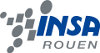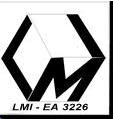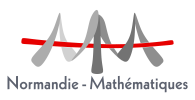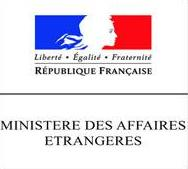Contact the webmaster